# Binary Coded Decimal

## What is BCD?

The BCD system offers relative ease of conversion between machine-readable and human-readable numerals.

## Why BCD?

With BCD, coders represent every decimal digit with four to eight digits, often called bits, using only zero and one. For example, you could represent a decimal value of five in BCD as 0101. The goal of BCD is to make it simpler to convert human numbers into values that a machine can understand.

An advantage of the Binary Coded Decimal system is each decimal digit is denoted by a group of 4 binary digits and that it allows easy conversion between decimal a base-10 system and binary a base-2 system.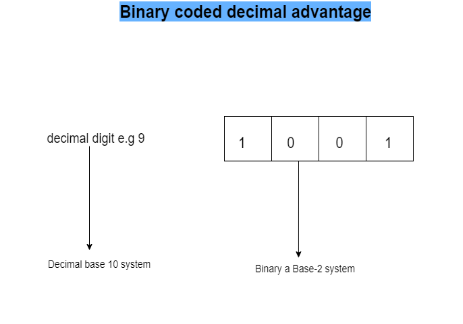A disadvantage is BCD code does not used all the states between binary 1010 for the decimal 10 and binary 1111 for the decimal 15.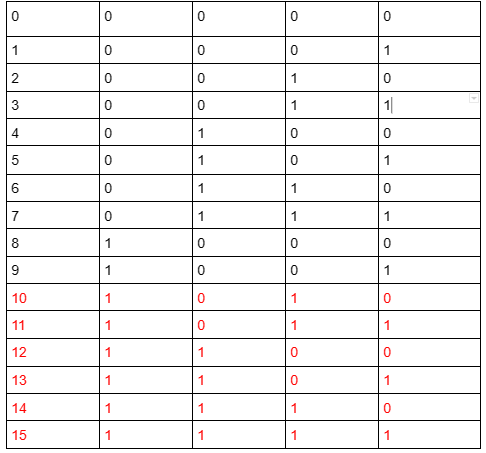## BCD Representation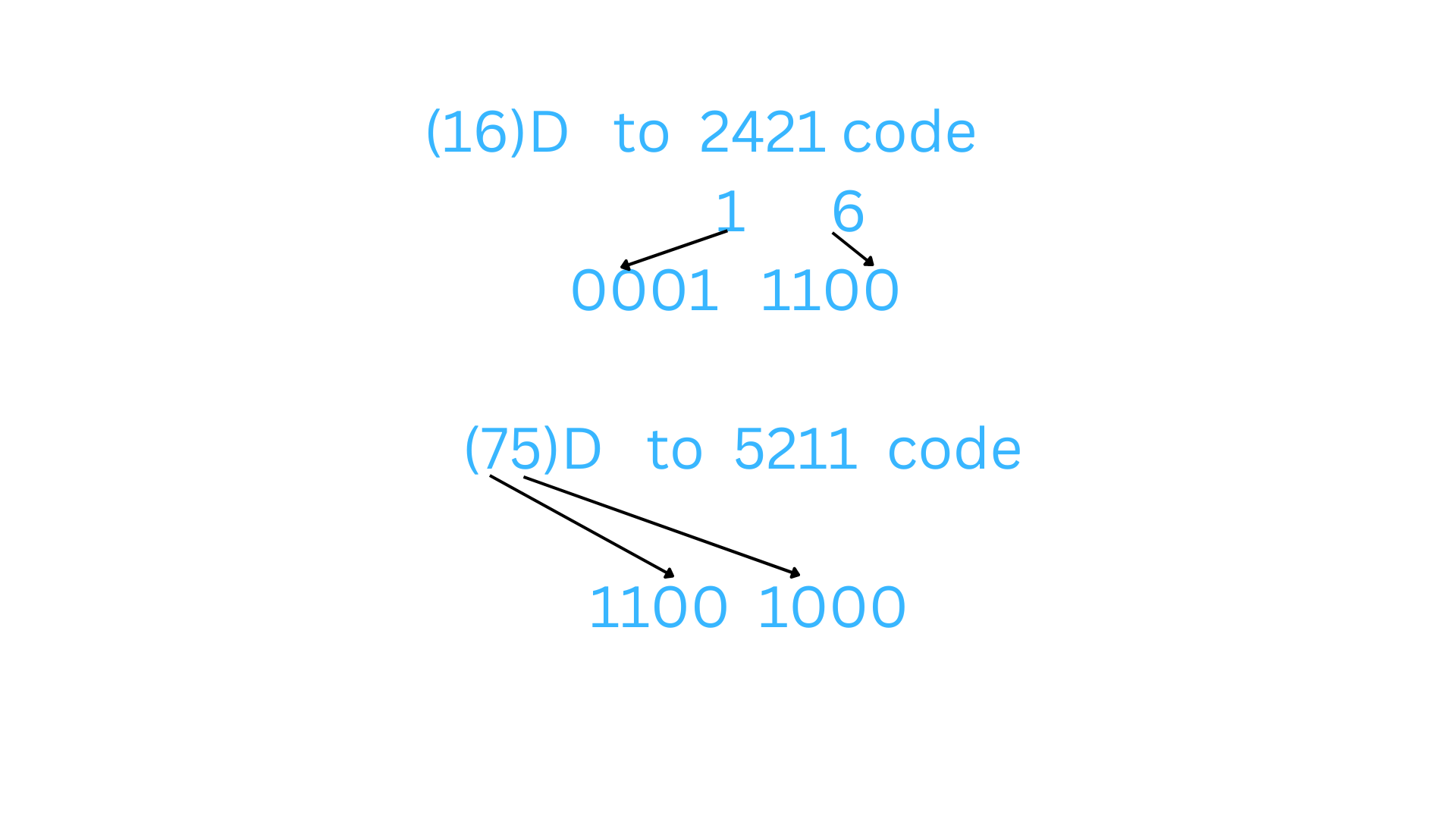## BCD Classification

BCD is classified into two categories weighted and non weighted codes.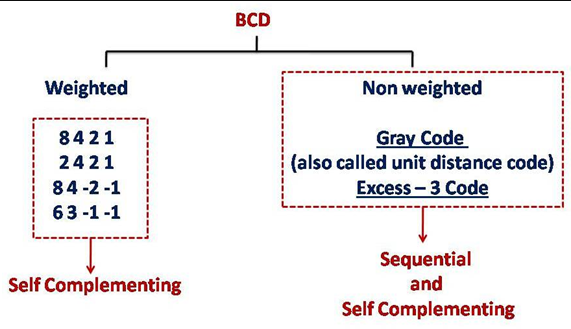## Weighted Binary Code

Weighted binary codes are those binary codes which obey the positional weight principle.

Each position of the number represents a specific weight. Several systems of the codes are used to express the decimal digits 0 through 9. In these codes each decimal digit is represented by a group of four bits.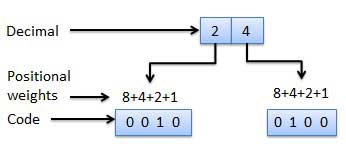Weighted codes are used in:

a) Data manipulation during arithmetic operation.

Data is manipulated by using the arithmetic instructions in digital computers. Data is manipulated to produce results necessary to give solution for the computation problems. The Addition, subtraction, multiplication and division are the four basic arithmetic operations.

b) For input/output operations in digital circuits

A digital I/O board is an interface board that adds the ability to input and output digital signals in parallel to a computer. Using a digital I/O device makes it possible to monitor (read) the statuses of measuring devices as well as the relays and operation switches of various types of control circuits. In addition to controlling output for lamps, LEDs, 7-segment displays, and relays, such products can also be used as an interface for digital communication with controllers such as a PLC (sequencer).c) To represent the decimal digits in calculators, volt meters etc.

8421 code

2421 code

5211 code

## Non-Weighted Codes

In this type of binary codes, the positional weights are not assigned. The examples of non-weighted codes are Excess-3 code and Gray code.

# Non-weighted Code Application

In Shift position encoding

Shift position encoding is a technique used in some non-weighted code applications, such as in text processing or natural language processing (NLP).

In the context of real-time applications, shift position encoding can be used in various scenarios, such as audio or video processing, where different data streams may have different delays or latencies. By aligning these data streams through shift position encoding, it is possible to improve the overall performance and accuracy of the application.

## BCD Usage in Alpha-Numeric Display and RTC

BCD was commonly used for displaying alpha-numeric in the past but in modern-day BCD is still used with real-time clocks or RTC chips to keep track of wall-clock time and it’s becoming more common for embedded microprocessors to include an RTC.

RTC (Real-Time Clock) is a hardware component that keeps track of the current date and time. BCD (Binary-Coded Decimal) is a way of representing decimal numbers in a binary format. Combining these two, an RTC application using BCD involves programming the RTC to keep track of the current date and time in BCD format.

## Alphanumeric Display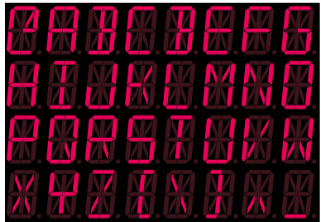It’s very common for RTCs to store the time in BCD format.

A binary clock might use LEDs to express binary values. With this clock each column of LEDs displays a binary-coded decimal numeral.

## BCD Format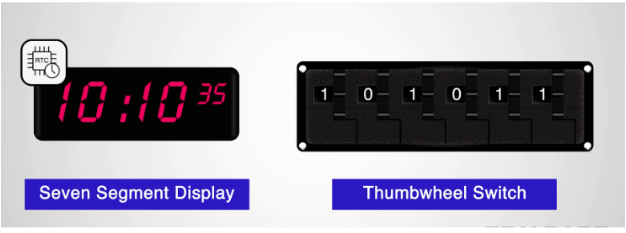## BCD to Excess-3 conversion

The process of converting BCD to Excess-3 is quite simple from other conversions. The Excess-3 code can be calculated by adding 3, i.e., 0011 to each four-digit BCD code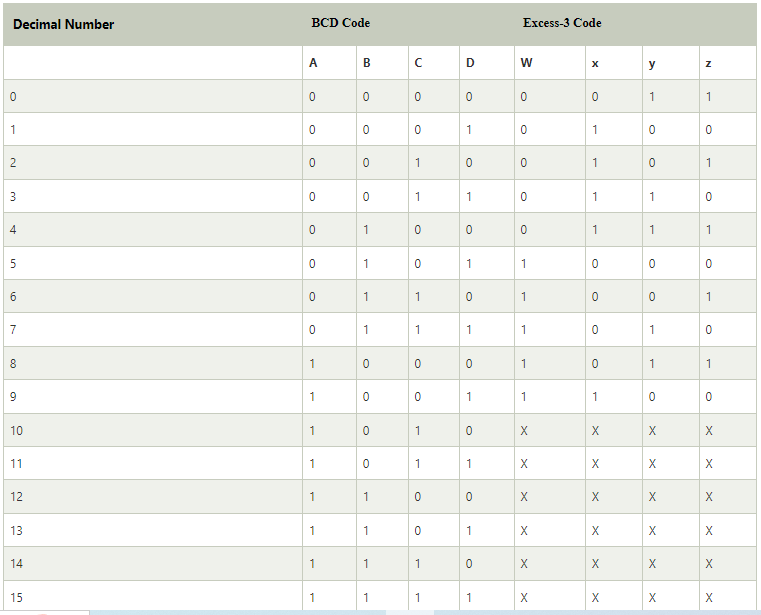Now, we will use the K-map method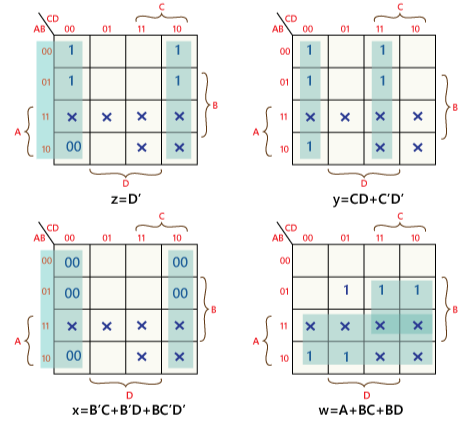w=A+BC+BD

x=B' C+B' D+BC' D'

y=CD+C'D'

z=D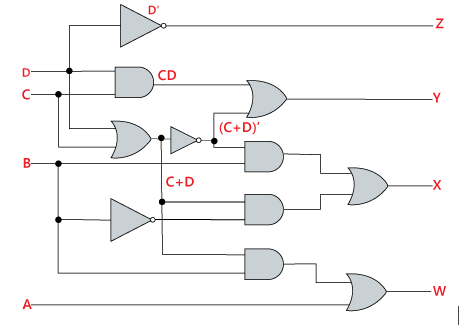### Example: (100001011001)BCD

To find the Excess-3 code of the given Excess-3 code, first, we will make the group of 4 bits from right to left. Then, we will add 0011 in each group of 4 bits in order to get the excess-3 code.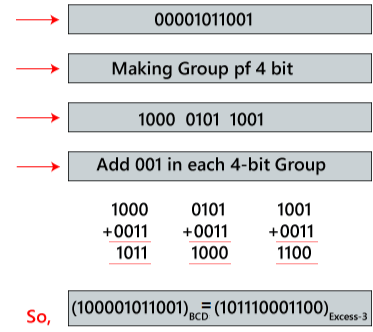## Excess-3 to BCD conversion

The process of converting Excess-3 to BCD is opposite to the process of converting BCD to Excess-3. The BCD code can be calculated by subtracting 3, i.e., 0011 from each four-digit Excess-3 code.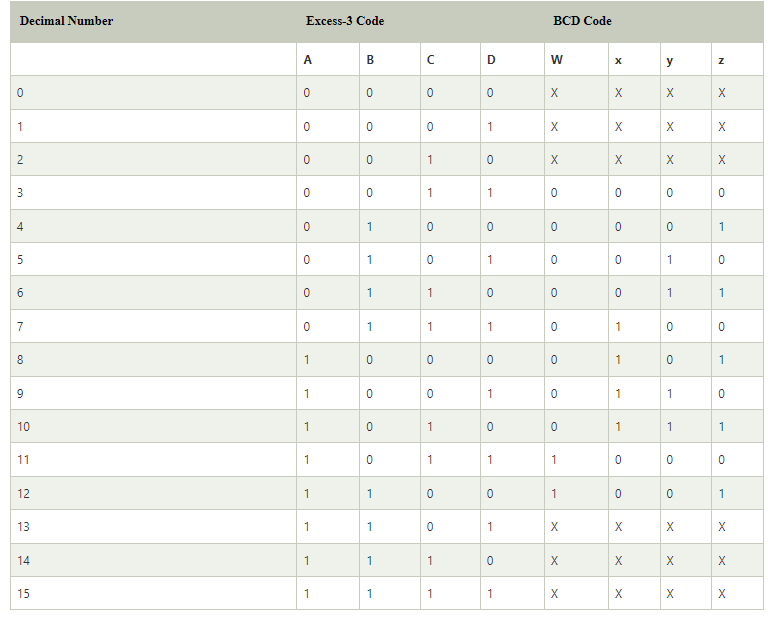on simplifying w=AB+ACD

B=x'y'+x'z'+xyz

C=y'z+yz'

D=z'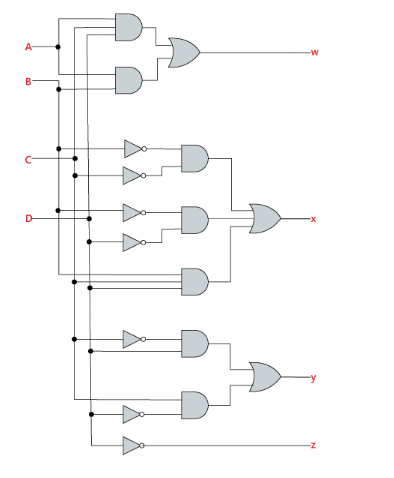## Example: (101110001100)Excess-3

To find the BCD code of the given BCD number, first, we make the group of 4 bits from right to left. Then, we subtract 0011 in each group of 4 bits in order to get the BCD code.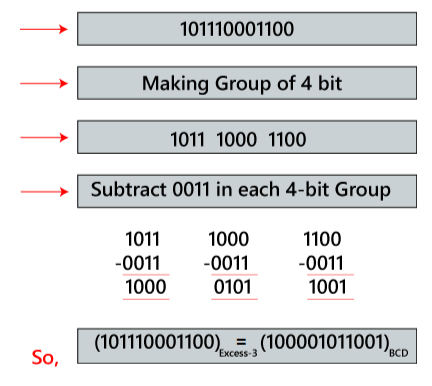## The procedure behind above solution*For 1011

*We have to subtract 1011 by 0011 (i.e by 3)

• Follow the 2's complement method

*For 2's complement first we have to take the 1's complement of 0011

• i.e, 1100

1100+ 1 =1101

We get output =1000

*For 1000

*We have to subtract 1000 by 0011 (i.e by 3)

*For 2's complement first we have to take the 1's complement of 0011

i.e, 1100

1100+ 1 =1101

We get output =0101

# For 1100

*We have to subtract 1100 by 0011 (i.e by 3)

*For 2's complement first we have to take the 1's complement of 0011

i.e, 1100

1100+ 1 =1101

We get output =1001

## 2421 Code

This code also a 4 bit application code where the binary weights carry 2, 4, 2, 1 from left to right.

DECIMAL NUMBER BINARY NUMBER 2421 CODE
0 0 0000
1 1 0001
2 10 0010
3 11 0011
4 100 0100
5 101 1011
6 110 1100
7 111 1101
8 1000 1110
9 1001 1111

## why 2421 code?

The weights of this code are 2, 4, 2 and 1.

This code has all positive weights. So, it is a positively weighted code.

It is an unnatural BCD code. Sum of weights of unnatural BCD codes is equal to 9.

It is a self-complementing code.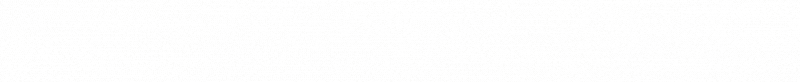Self-complementing codes provide the 9’s complement of a decimal number, just by interchanging 1’s and 0’s in its equivalent 2421 representation.

## Example

Let us find the 2421 equivalent of the decimal number 786. This number has 3 decimal digits 7, 8 and 6. From the table, we can write the 2421 codes of 7, 8 and 6 are 1101, 1110 and 1100 respectively.

Therefore, the 2421 equivalent of the decimal number 786 is 110111101100.

# 5211 Code

This code is also a 4 bit application code where the binary weights carry 5, 2, 1, 1 from left to right.

DECIMAL NUMBER BINARY NUMBER 5211 CODE
0 0 0000
1 1 0001
2 10 0011
3 11 0101
4 100 0111
5 101 1000
6 110 1010
7 111 1100
8 1000 1110
9 1001 1111

## why 5211 Code?

Because, 5211 is reflective

A code is said to be reflective when code for 9 is complement for the code for 0, and so is for 8 and 1 codes, 7 and 2, 6 and 3, 5 and 4. In the above table

• "9" is the 1's complement of "0".

• "8" is the complement of "1".

• "7" is the complement of "2" etc..

# Sequential Codes

Sequential codes are the codes in which 2 subsequent numbers in binary representation differ by only one digit.

DECIMAL NUMBER BINARY NUMBER 8421 CODE EXCESS-3
0 0 0000 0011
1 1 0001 0100
2 10 0010 0101
3 11 0011 0110
4 100 0100 0111
5 101 0101 1000
6 110 0110 1001
7 111 0111 1010
8 1000 1000 1011
9 1001 1001 1100